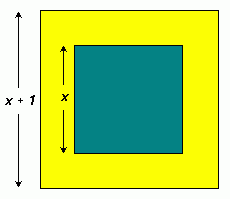SEARCH HOMEMath Central Quandaries & QueriesQuestion from Maria, a student: i have a problem with this quadratic word problem which i am trying to solve it but couldn,t get it please help me to solve this Two concentric circles are drawn, the radius of one being 2cm greater than that of the other. The area of the ring enclosed between the two circles is one quarter of the area of the smaller circle.Calculate the radii of the circles,correct to three significant figures. (Don,t substitute for pie)Hi Maria.

The area of a circle is based on its radius - I am sure you know the formula for that, so I will solve a parallel problem for you to show you how to go about solving your own question.

My problem: One green square is inside a yellow square as shown below.The area of the green square is one third the area of the yellow border. The side length of the yellow square is 1 unit more than the side length of the green square.

What is the side length of each square?

Solution:

Let Y = area of the yellow square and G = area of the green square.

Then Y - G = the area of the yellow border.

Since the area of a square is just the square of the side length, we know
Y = (x + 1)2 and G = x2.

But we are also told that the area of the green square (G) is one third the area of the yellow border (Y-G), so:

G = (1 /3 )(Y-G), hence

x2 = (1 /3 ) ( (x + 1)2 - x2 )

3x2 = ( x2 + 2x + 1 - x2 )
3x2 = 2x + 1
3x2 - 2x - 1 = 0
(3x + 1)(x - 1) = 0

This gives two answers, but one of them is negative, which doesn't fit the idea of a side length, so we can ignore it. Therefore x = 1, so the green square has sides of 1 unit each, and the yellow square has sides of 2 (that's x + 1) units each.

In this case, I needed to solve a quadratic equation by factoring, but I could have used the quadratic formula if I couldn't easily factor it.

When you use this method for your concentric circles, you'll get the right answer to your question.

Hope this helps,
Stephen La Rocque.Math Central is supported by the University of Regina and The Pacific Institute for the Mathematical Sciences.